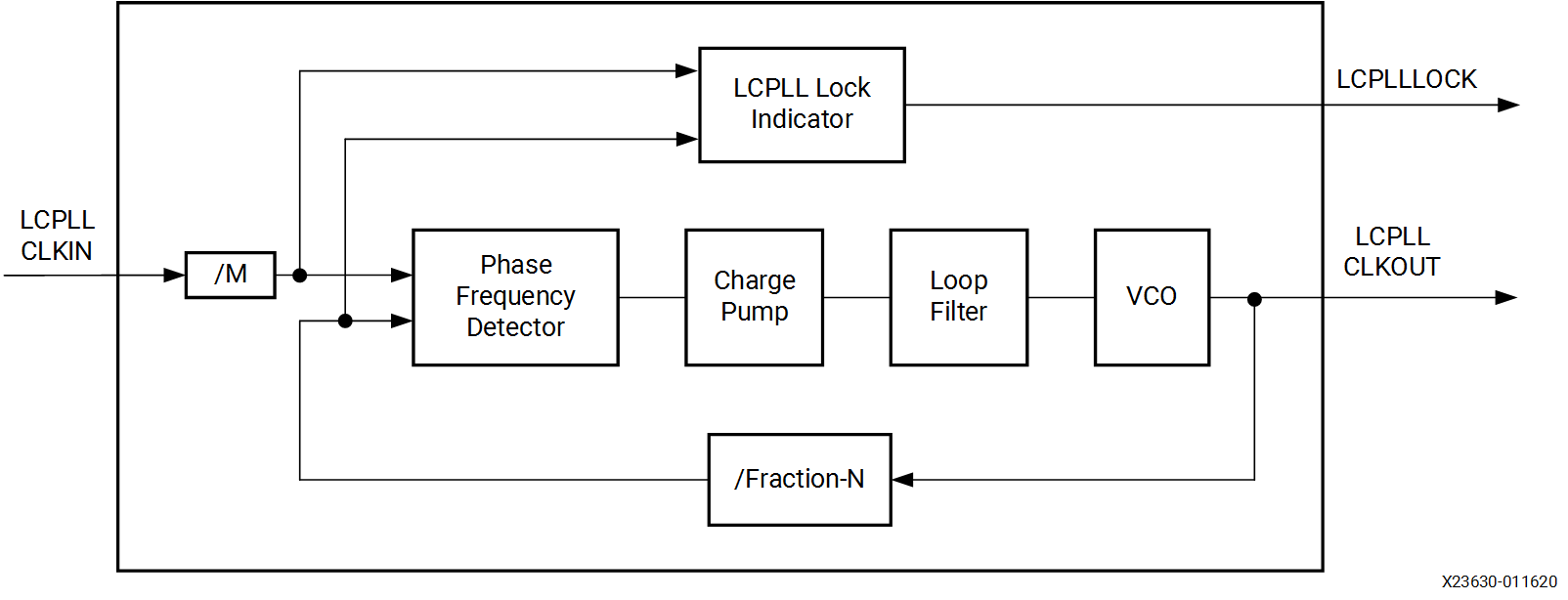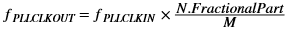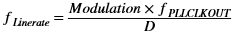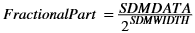# LC Tank PLL

## Versal ACAP GTM Transceivers Architecture Manual (AM017)

Document ID
AM017
Release Date
2022-04-27
Revision
1.0 English

Each GTM Quad contains two LC-based PLLs, one per HSCLK block. The LCPLL input reference clock selection is described in Reference Clock Selection and Distribution. The LCPLL outputs feed the TX and RX clock dividers of each serial transceiver channel, which then controls the generator of serial and parallel clocks used by the PMA and PCS blocks.

The following figure illustrates a conceptual view of the LCPLL architecture. The input clock is divided by a factor of M before it is fed into the phase frequency detector. The feedback divider N determines the VCO multiplication ratio. The fractional-N divider is supported where the effective ratio is a combination of the N factor plus a fractional part. Fractional-N divider performance is pending characterization. The LCPLL output frequency depends on the setting of LCPLLCLKOUT_RATE. When LCPLLCLKOUT_RATE is set to HALF, the output frequency is half of the VCO frequency. A lock indicator block compares the frequency of the reference clock and the VCO feedback clock to determine if a frequency lock has been achieved.

Figure 1. LCPLL Block DiagramThe LCPLL in the GTM transceiver has a nominal operating range between 9.5 GHz to 15 GHz. For additional information regarding the exact LCPLL operating range for different device speed grades, refer to the specific Versal ACAP data sheet. The Versal ACAP Transceivers Wizard chooses the appropriate LCPLL settings based on application requirements.

The following equation shows how to determine the LCPLL output frequency (GHz).

Figure 2. Determine the LCPLL Output FrequencyThe following equation shows how to determine the line rate (Gb/s).

Figure 3. Determine the Line RateThe following equation shows how to determine the fractional part of the feedback divider presented in Figure 2.

Figure 4. Determine the Fractional Part of the Feedback DividerTable 1. LCPLL Divider Settings
Factor Attribute/Port Valid Settings
M HSCLK[0/1]_LCPLL_CFG0 1, 2, 3, 4
N A_HS[0/1]_LCPLLFBDIV The valid divider range is 16–160
D TXOUT_DIV

RXOUT_DIV

1, 2, 4
Modulation See TX Configurable Driver 4 (NRZ), 8 (PAM4)
SDMWIDTH HSCLK[0/1]_LCPLL_LGC_CFG1 12, 16, 20, 24
SDMDATA HSCLK[0/1]_LCPLLSDMDATA

[SDMWIDTH + 1:SDMWIDTH]: two's complement integer in range [–2, 1]

[SDMWIDTH – 1:0]: fractional part in range [0, (2SDMWIDTH – 1)]